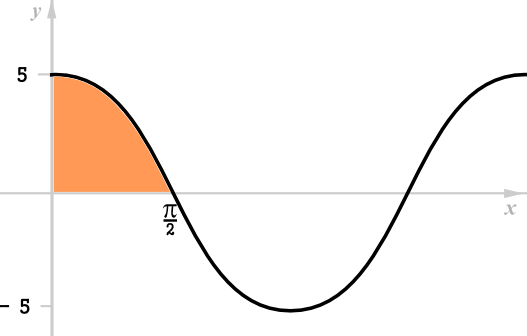# Exam-Style Questions.

## Problems adapted from questions set for previous Mathematics exams.

### 1.

IB Standard

Find the value of $$a$$ if $$\pi \lt a \lt 2\pi$$ and:

$$\int_\pi^a sin3x dx = -\frac13$$

### 2.

IB StandardThis graph represents the function $$f:x\to a \cos x, a\in \mathbf N$$

(a) Find the value of $$a$$.

(b) Find the area of the shaded region.

### 3.

IB Standard

Find $$f(x)$$ if $$f'(x)=6 \sin2x$$ and the graph of $$f(x)$$ passes through the point $$(\frac{\pi}{3},11)$$.

If you would like space on the right of the question to write out the solution try this Thinning Feature. It will collapse the text into the left half of your screen but large diagrams will remain unchanged.

The exam-style questions appearing on this site are based on those set in previous examinations (or sample assessment papers for future examinations) by the major examination boards. The wording, diagrams and figures used in these questions have been changed from the originals so that students can have fresh, relevant problem solving practice even if they have previously worked through the related exam paper.

The solutions to the questions on this website are only available to those who have a Transum Subscription.

Exam-Style Questions Main Page

Search for exam-style questions containing a particular word or phrase:

To search the entire Transum website use the search box in the grey area below.